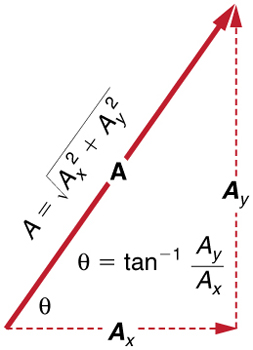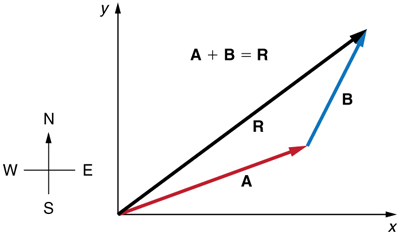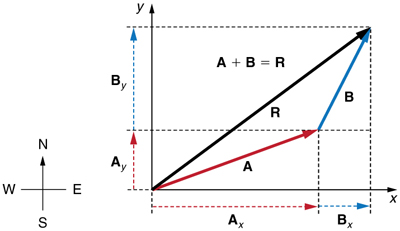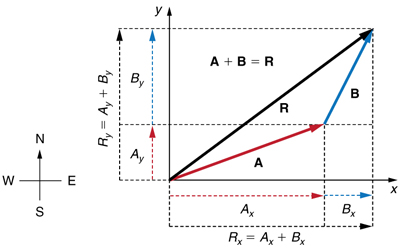# 3.3 Vector addition and subtraction: analytical methods  (Page 2/5)

 Page 2 / 5
$A=\sqrt{{A}_{{x}^{2}}+{A}_{{y}^{2}}}$
$\theta ={\text{tan}}^{-1}\left({A}_{y}/{A}_{x}\right)\text{.}$The magnitude and direction of the resultant vector can be determined once the horizontal and vertical components A x size 12{A rSub { size 8{x} } } {} and A y size 12{A rSub { size 8{y} } } {} have been determined.

Note that the equation $A=\sqrt{{A}_{x}^{2}+{A}_{y}^{2}}$ is just the Pythagorean theorem relating the legs of a right triangle to the length of the hypotenuse. For example, if ${A}_{x}$ and ${A}_{y}$ are 9 and 5 blocks, respectively, then $A=\sqrt{{9}^{2}{\text{+5}}^{2}}\text{=10}\text{.}3$ blocks, again consistent with the example of the person walking in a city. Finally, the direction is $\theta ={\text{tan}}^{–1}\left(\text{5/9}\right)=29.1º$ , as before.

## Determining vectors and vector components with analytical methods

Equations ${A}_{x}=A\phantom{\rule{0.25em}{0ex}}\text{cos}\phantom{\rule{0.25em}{0ex}}\theta$ and ${A}_{y}=A\phantom{\rule{0.25em}{0ex}}\text{sin}\phantom{\rule{0.25em}{0ex}}\theta$ are used to find the perpendicular components of a vector—that is, to go from $A$ and $\theta$ to ${A}_{x}$ and ${A}_{y}$ . Equations $A=\sqrt{{A}_{x}^{2}+{A}_{y}^{2}}$ and $\theta ={\text{tan}}^{\text{–1}}\left({A}_{y}/{A}_{x}\right)$ are used to find a vector from its perpendicular components—that is, to go from ${A}_{x}$ and ${A}_{y}$ to $A$ and $\theta$ . Both processes are crucial to analytical methods of vector addition and subtraction.

## Adding vectors using analytical methods

To see how to add vectors using perpendicular components, consider [link] , in which the vectors $\mathbf{A}$ and $\mathbf{B}$ are added to produce the resultant $\mathbf{R}$ .Vectors A size 12{A} {} and B size 12{B} {} are two legs of a walk, and R size 12{R} {} is the resultant or total displacement. You can use analytical methods to determine the magnitude and direction of R size 12{R} {} .

If $\mathbf{A}$ and $\mathbf{B}$ represent two legs of a walk (two displacements), then $\mathbf{R}$ is the total displacement. The person taking the walk ends up at the tip of $\mathbf{R}.$ There are many ways to arrive at the same point. In particular, the person could have walked first in the x -direction and then in the y -direction. Those paths are the x - and y -components of the resultant, ${\mathbf{R}}_{x}$ and ${\mathbf{R}}_{y}$ . If we know ${\mathbf{\text{R}}}_{x}$ and ${\mathbf{R}}_{y}$ , we can find $R$ and $\theta$ using the equations $A=\sqrt{{{A}_{x}}^{2}+{{A}_{y}}^{2}}$ and $\theta ={\text{tan}}^{–1}\left({A}_{y}/{A}_{x}\right)$ . When you use the analytical method of vector addition, you can determine the components or the magnitude and direction of a vector.

Step 1. Identify the x- and y-axes that will be used in the problem. Then, find the components of each vector to be added along the chosen perpendicular axes . Use the equations ${A}_{x}=A\phantom{\rule{0.25em}{0ex}}\text{cos}\phantom{\rule{0.25em}{0ex}}\theta$ and ${A}_{y}=A\phantom{\rule{0.25em}{0ex}}\text{sin}\phantom{\rule{0.25em}{0ex}}\theta$ to find the components. In [link] , these components are ${A}_{x}$ , ${A}_{y}$ , ${B}_{x}$ , and ${B}_{y}$ . The angles that vectors $\mathbf{A}$ and $\mathbf{B}$ make with the x -axis are ${\theta }_{\text{A}}$ and ${\theta }_{\text{B}}$ , respectively.To add vectors A size 12{A} {} and B size 12{B} {} , first determine the horizontal and vertical components of each vector. These are the dotted vectors A x size 12{A rSub { size 8{x} } } {} , A y size 12{A rSub { size 8{y} } } {} , B x size 12{B rSub { size 8{x} } } {} and B y size 12{B rSub { size 8{y} } } {} shown in the image.

Step 2. Find the components of the resultant along each axis by adding the components of the individual vectors along that axis . That is, as shown in [link] ,

${R}_{x}={A}_{x}+{B}_{x}$

and

${R}_{y}={A}_{y}+{B}_{y}\text{.}$The magnitude of the vectors A x size 12{A rSub { size 8{x} } } {} and B x size 12{B rSub { size 8{x} } } {} add to give the magnitude R x size 12{R rSub { size 8{x} } } {} of the resultant vector in the horizontal direction. Similarly, the magnitudes of the vectors A y size 12{A rSub { size 8{y} } } {} and B y size 12{B rSub { size 8{y} } } {} add to give the magnitude R y size 12{R rSub { size 8{y} } } {} of the resultant vector in the vertical direction.

Components along the same axis, say the x -axis, are vectors along the same line and, thus, can be added to one another like ordinary numbers. The same is true for components along the y -axis. (For example, a 9-block eastward walk could be taken in two legs, the first 3 blocks east and the second 6 blocks east, for a total of 9, because they are along the same direction.) So resolving vectors into components along common axes makes it easier to add them. Now that the components of $\mathbf{R}$ are known, its magnitude and direction can be found.

Is the force attractive or repulsive between the hot and neutral lines hung from power poles? Why?
what's electromagnetic induction
electromagnetic induction is a process in which conductor is put in a particular position and magnetic field keeps varying.
Lukman
wow great
Salaudeen
what is mutual induction?
je
mutual induction can be define as the current flowing in one coil that induces a voltage in an adjacent coil.
Johnson
how to undergo polarization
show that a particle moving under the influence of an attractive force mu/y³ towards the axis x. show that if it be projected from the point (0,k) with the component velocities U and V parallel to the axis of x and y, it will not strike the axis of x unless u>v²k² and distance uk²/√u-vk as origin
show that a particle moving under the influence of an attractive force mu/y^3 towards the axis x. show that if it be projected from the point (0,k) with the component velocities U and V parallel to the axis of x and y, it will not strike the axis of x unless u>v^2k^2 and distance uk^2/√u-k as origin
No idea.... Are you even sure this question exist?
Mavis
I can't even understand the question
yes it was an assignment question "^"represent raise to power pls
Gabriel
Gabriel
An engineer builds two simple pendula. Both are suspended from small wires secured to the ceiling of a room. Each pendulum hovers 2 cm above the floor. Pendulum 1 has a bob with a mass of 10kg . Pendulum 2 has a bob with a mass of 100 kg . Describe how the motion of the pendula will differ if the bobs are both displaced by 12º .
no ideas
Augstine
if u at an angle of 12 degrees their period will be same so as their velocity, that means they both move simultaneously since both both hovers at same length meaning they have the same length
Modern cars are made of materials that make them collapsible upon collision. Explain using physics concept (Force and impulse), how these car designs help with the safety of passengers.
calculate the force due to surface tension required to support a column liquid in a capillary tube 5mm. If the capillary tube is dipped into a beaker of water
find the time required for a train Half a Kilometre long to cross a bridge almost kilometre long racing at 100km/h
method of polarization
Ajayi
What is atomic number?
The number of protons in the nucleus of an atom
Deborah
type of thermodynamics
oxygen gas contained in a ccylinder of volume has a temp of 300k and pressure 2.5×10Nm
why the satellite does not drop to the earth explain
what is a matter
Yinka
what is matter
Yinka
what is matter
Yinka
what is a matter
Yinka
I want the nuclear physics conversation
Mohamed
because space is a vacuum and anything outside the earth 🌎 can not come back without an act of force applied to it to leave the vacuum and fall down to the earth with a maximum force length of 30kcm per second
Clara
at t=0second,aparticles moving in x-y plain with aconstant acceleration has avelocity of initial velocity =(3i-2j)m/s and is at the origion.at t=3second the particle's velocity is final velocity=(9i+7j)then how to find the acceleration?
how about the formula like v^2=u^2+2as
Bayuo
a=v-u/t
Doreen
what is physics
Yinka
why is there a maximum distance at which the image can exist behind a convex mirror
The ball of a simple pendulum take 0.255 to swing from its equilibrium position to one extreme. Calculate it period.
The Ball of a simple pendulum take 0.255 to swing from its equilibrium position to one extreme. calculate its period
Abubakr
why is there a maximum distance at which the image can exist behind a convex mirror
Alfred
amplitude=0 .255s period=4×.255=1.02 sec period is one complete cycle
MUKHTAR

#### Get Jobilize Job Search Mobile App in your pocket Now!ByByBy Candice ButtsByBy Tod McGrathBy Lakeima RobertsBy OpenStaxBy Brooke DelaneyBy Anonymous UserBy Janet ForresterBy OpenStaxBy Sheila Lopez